Courses

# Chemistry - 2014 Past Year Paper

## 35 Questions MCQ Test IIT JAM Chemistry Past Year Papers | Chemistry - 2014 Past Year Paper

Description
This mock test of Chemistry - 2014 Past Year Paper for IIT JAM helps you for every IIT JAM entrance exam. This contains 35 Multiple Choice Questions for IIT JAM Chemistry - 2014 Past Year Paper (mcq) to study with solutions a complete question bank. The solved questions answers in this Chemistry - 2014 Past Year Paper quiz give you a good mix of easy questions and tough questions. IIT JAM students definitely take this Chemistry - 2014 Past Year Paper exercise for a better result in the exam. You can find other Chemistry - 2014 Past Year Paper extra questions, long questions & short questions for IIT JAM on EduRev as well by searching above.
QUESTION: 1

Solution:
QUESTION: 2

Solution:
QUESTION: 3

### The carbonyl stretching frequency (VC = O) is highest for:

Solution:
QUESTION: 4

The homolytic breaking of the Ca – Cb bond is easiest in:

Solution:
QUESTION: 5

Tollen’s test will be negative for:

Solution:
QUESTION: 6

Which one among the following is a sesquiterpene?

Solution:
QUESTION: 7

The predicted geometry of TeF4 by VSEPR theory is:

Solution:
QUESTION: 8

Among the following, the isoelectric pair is:

Solution:
QUESTION: 9

The metal ion of an enzyme involved in hydration of CO2 is:

Solution:
QUESTION: 10

Among the following, the element having maximum inert pair effect is: [Given : Atomic number of Ge = 30, Pb = 82, Si = 14 and Sn = 50]

Solution:
QUESTION: 11

The reactivity of compounds I- IV with maleic anhydride (V) follows the order: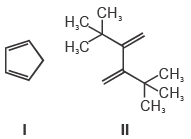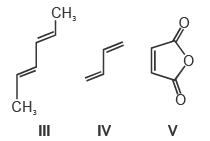Solution:
QUESTION: 12

Which one among the following molecules is chiral?

Solution:
QUESTION: 13

Identify the starting material I in the given reaction: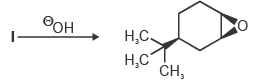Solution:
QUESTION: 14

The major product for the following reaction is: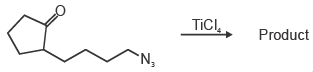Solution:
QUESTION: 15

The structure of the major product in the following reaction is: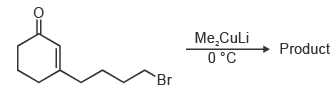Solution:
QUESTION: 16

The correct orientation of dipoles in pyrrole and pyridine is:

Solution:
QUESTION: 17

Specific rotations of freshly prepared aqueous solutions of I and II are +112 and +18.7, respectively. On standing, the optical rotation of aqueous solution of I slowly decreases to give a final value of +52.7 due to equilibration with II. Under this state of equilibrium, what is the ratio II : I?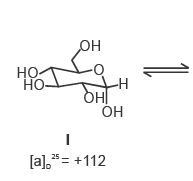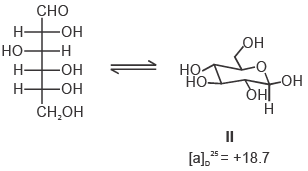Solution:
QUESTION: 18

The major product formed in the following reaction is :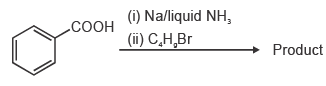Solution:
QUESTION: 19

In boron neutron capture therapy, the initial boron isotope used and the particle generated after neutron capture respectively are:

Solution:
QUESTION: 20

The number of a and b particle(s), generated in the following radioactive decay process, are: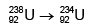Solution:
QUESTION: 21

In the measurement of hardness of water by complexometric titration, identify P and Q in the following equation.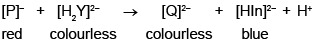Solution:
QUESTION: 22

An aqueous solution of hemoglobin has a molar absorptivity value of 18,600 L mol–1 cm–1 for an absorbance value of 0.1 at 540 nm (Given : cell thickness = 1 cm). The concentration (in μM) of the hemoglobin solution is

Solution:
QUESTION: 23

The electronic transitions responsible for the colour of K2Cr2O7 and porphine in their solid state respectively are:

Solution:
QUESTION: 24

The correct order of M – C (M = Ti, V, Cr and Mn) bond stretching frequency is: (Given : Atomic number of Ti = 22, V = 23, Cr = 24 and Mn = 25)

Solution:
QUESTION: 25

For the following reactions, the metal complexes X and Y are: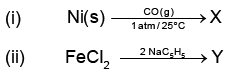Solution:
QUESTION: 26

The correct order of crystal field strength is:
(Given: en = ethylenediamine)

Solution:
QUESTION: 27

The carbon-oxygen bond in an organic compound absorbs electromagnetic radiation of frequency 6 × 1013 Hz. This frequency corresponds to the region:

Solution:
QUESTION: 28

According to the equipartition principle of energy, the molar heat capacity at constant volume for CO2(g), SO2(g) and H2O(g) follows the trend:

Solution:
QUESTION: 29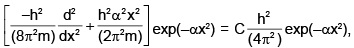where h, π, m and α are constants. Then C is:

Solution:
QUESTION: 30

Among Ar. NH4Cl, HF and HCI, the strength of interatomic/intermolecular forces follows the order:

Solution:
QUESTION: 31

The number of degrees of freedom of freedom in the homogeneous liquid region of a two component system with a eutectic point, at one atmosphere pressure, is:

Solution:
QUESTION: 32

The ionic strength of 0.1 M aqueous solution of Fe2(SO4)3 is:

Solution:
QUESTION: 33

If the transport number of Na+ is 0.463 (dilute solution of NaCl in methanol), the transport number of H+ (dilute solution of HCI in methanol) is: (Given,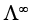(NaCl in methanol) = 96.9 ohm-1 cm2 mol-1 and(HCI in methanol) = 192 ohm-1 cm2mol–1)

Solution:
QUESTION: 34

Charcoal (1 gram) of surface area 100 m2 per gram, absorbs 60 mg of acetic acid from an aqueous solution at 25°C and 1 atmosphere pressure. The number of moles of acetic acid adsorbed per cm2 of charcoal surface is:

Solution:
QUESTION: 35

The change in entropy for the following transformations is respectively: (+ indicates increase, – indicates decrease and 0 indicates no change)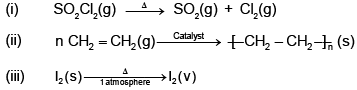(iv) Adiabatic reversible expansion of an ideal gas

Solution: